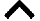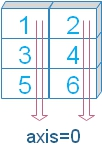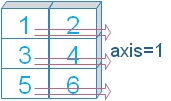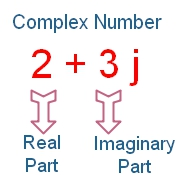# Numpy Array Methods

We can use these methods to get different outputs from array.

 `all` Test all elements in given axis is True or not any Test all elements in given axis is True or not argmax Position of Max value along any axis argmin Position of Min value along any axis argsort Return the indices after sorting choose Select elements based on position clip Set a maximum and minimum values for elements compress Selected elements based on condition copy Copy one array to another array cumprod Cumulative product of elements cumsum Cumulative sum of elements diagonal Cumulative sum of elements imag Imaginary numbers from main array amax Highest number in the array mean Arithmetic mean along an axis min Arithmetic min along an axis nonzero Returns array of position of Non zero elements partition Elements sorted in order based on nth position element

## all

Check if all elements are True in a given axis ( optional )
``````import numpy as my_np
my_array=my_np.array([1,4,5]) # change the elements to see result.
print(my_np.all(my_array)) # True``````
`axis` Try two dimensional array, we will use axis to specify AND operation in different axis.
``````my_array = my_np.array([[1,2],[3,False],[4,True],[5,False]])
print(my_np.all(my_array,axis=0))``````
Output
``[ True False]``
`out` We can place the result in another array.
`keepdims`
``````my_array = my_np.array([[1,2],[3,False],[4,True],[5,False]])
print(my_np.all(my_array,axis=0,keepdims=True))``````
Output is here
``[[ True False]]``

## anyCheck whether array elements evaluates to an array. Returns True or false.
``````import numpy as my_np
my_array = my_np.array([[1,2],[3,False],[4,True],[5,False]])
print(my_np.any(my_array))``````
Output is here
``True``
`axis`
``print(my_np.any(my_array,axis=0))``
Output
``[ True  True]``
`keepdims`
``print(my_np.any(my_array,axis=0,keepdims=True))``
Output
``[[ True  True]]``

## argmaxIndices of the maximum value along with an axis ( optional )
``````import numpy as my_np
my_array = my_np.array([4,3,7,0,1])
print(my_np.argmax(my_array))  # 2
my_array = my_np.array([[1,5],[3,4],[9,True],[5,False]])
print(my_np.argmax(my_array))  # 4 ``````
`axis` Optional
``````import numpy as my_np
my_array = my_np.array([[1,5],[3,4],[4,True],[5,False]])
print(my_np.argmax(my_array,axis=0)) # [3 0]``````
`out` Output should be stored in a defined array of matching shape and dtype.
``````import numpy as my_np
my_array = my_np.array([[1,5],[3,4],[4,True],[5,False]])
my_array2=my_np.array([0,0])
my_np.argmax(my_array,axis=0,out=my_array2)
print(my_array2) # [3 0]``````
Using a three dimensional array
``````import numpy as my_np
my_array = my_np.array([[[ 30,  1,  2],[ 3,  50,  5],[ 6,  7,  8]],
[[ 9, 10, 11],[32, 13, 48],[15, 16, 17]],
[[18, 19, 20],[21, 47, 23],[36, 25, 26]]])
print(my_array.argmax())
print(my_array.argmax(axis=0))``````
Output is here
``````4
[[0 2 2]
[1 0 1]
[2 2 2]]``````

## argminIndices of the minimum value along with an axis ( optional )
``````import numpy as my_np
my_array = my_np.array([4,3,7,0,1])
print(my_np.argmin(my_array))  # 3
my_array = my_np.array([[1,5],[3,4],[9,True],[5,False]])
print(my_np.argmin(my_array))  # 7 ``````
`axis` Optional
``````import numpy as my_np
my_array = my_np.array([[1,5],[3,4],[4,True],[5,False]])
print(my_np.argmin(my_array,axis=0)) # [0 3]``````
`out` Optional, Result will be stored in this array. If given it must have the same shape and dtype.
``````import numpy as my_np
my_array = my_np.array([[1,5],[3,4],[4,True],[5,False]])
my_array2=my_np.array([0,0])
my_np.argmin(my_array,axis=0,out=my_array2)
print(my_array2) # [0 3]``````

## argsortSort in given axis ( optional ) and returns an array of indices after sorting.
``````import numpy as my_np
my_array = my_np.array([6,4,5,8,3])
print(my_np.argsort(my_array)) # [4 1 2 0 3]``````
``````import numpy as my_np
my_array = my_np.array([[6,4],[5,8],[3,7]])
print(my_np.argsort(my_array))``````
output
``````[[1 0]
[0 1]
[0 1]]``````
`axis`(optional)
``````import numpy as my_np
my_array = my_np.array([[6,4],[5,8],[3,7]])
print(my_np.argsort(my_array,axis=0))``````
Output
``````[[2 0]
[1 2]
[0 1]]``````
`kind` ( optional ) type of sort algorithm, values are 'quicksort', 'mergesort', 'heapsort'
``````import numpy as my_np
my_array = my_np.array([[6,4],[5,8],[3,7]])
print(my_np.argsort(my_array,kind='heapsort'))``````
`order` (Optional), When fields are defined then given field is considered first for sorting. After sorting input field, rest of the fields are used.
``````import numpy as my_np
my_array = my_np.array([('Alex',80,50.6),('Ronal',50,60.3),('Jack',55,72.3)],
dtype=[('name', 'U10'), ('mark', 'i4'), ('average', 'f4')])
print(my_np.argsort(my_array,order='name')) # [0 2 1]
print(my_np.argsort(my_array,order='mark')) # [1 2 0]
print(my_np.argsort(my_array,order='average')) # [0 1 2]``````

## chooseSelect elements based on input positions. `choices` array and it must be broadcastable
``````import numpy as my_np
my_array = [[0, 5,7, 3], [19, 15, 12, 14],[27, 23, 21, 29], [39, 33, 38, 34]]
ch=[2,3,1,0]
print(my_np.choose(ch, my_array)) # [27 33 12 3]``````
`mode` (Optional ) value can be raise( default ), clip,wrap
clip : If value is more than n-1 then it is changed to n-1.
wrap : Math module is used to keep the value in range of n-1.
Check the example to know how mode is used
``````import numpy as my_np
my_array = [[0, 5,7, 3], [19, 15, 12, 14],[27, 23, 21, 29], [39, 33, 38, 34]]
ch=[2,3,1,0]
print(my_np.choose(ch, my_array)) # [27 33 12 3]
ch=[2,4,1,0]
print(my_np.choose(ch, my_array,mode='clip')) # [27 33 12 3]
print(my_np.choose(ch, my_array,mode='wrap')) # [27 5 12 3] # 4 mod 4 is 0
ch=[2,5,1,0]
print(my_np.choose(ch, my_array,mode='wrap')) # [27 15 12 3] # 5 mod 4 is 1``````

## clipElements are restricted to a maximum and minimum value.
Here minimum value is given as 10 , no maximum value ( None )
``````import numpy as my_np
my_array = [5,12,6,9,17]
print(my_np.clip(my_array,10,None)) # [10 12 10 10 17]``````
Maximum value is there, no minimum value
``````import numpy as my_np
my_array = [5,12,6,9,17]
print(my_np.clip(my_array,None,10)) # [ 5 10  6  9 10]``````
Both Maximum and Minimum value is given
``````import numpy as my_np
my_array = [5,12,6,9,17]
print(my_np.clip(my_array,10,12)) # [10 12 10 10 12]``````
`out` Optional : Matching array to store the result ( output )
``````import numpy as my_np
my_array = [5,12,6,9,17]
my_out=my_np.array([0,0,0,0,0])
my_np.clip(my_array,10,12,out=my_out)
print(my_out) # [10 12 10 10 12]``````

## compressReturns an array with elements matching to the given pattern.
Here axis is not given so the condition is applied on the flattened array.
``````import numpy as my_np
my_array = my_np.array([[1,5],[3,4],[4,3],[5,7]])
print(my_np.compress([0,1,1,1,0,1],my_array)) # [5 3 4 3]``````
`axis` Optional along the axis
``````import numpy as my_np
my_array = my_np.array([[1,5],[3,4],[4,3],[5,7]])
print(my_np.compress([0,1,1],my_array,axis=0)) ``````
``````[[3 4]
[4 3]]``````
`out` Optional : Matching array to store the output ( result )
``````import numpy as my_np
my_array = my_np.array([[1,5],[3,4],[4,3],[5,7]])
my_out=my_np.array([[0,0],[0,0]])
my_np.compress([0,1,1],my_array,axis=0,out=my_out)
print(my_out)``````
Output is here
``````[[3 4]
[4 3]]``````

## copycopy array
``````import numpy as my_np
my_array = my_np.array([1,2,3,4])
my_array2=my_array.copy()
print(my_array2) # [1 2 3 4]``````

## cumprodCumulative product of elements of array.
``````import numpy as my_np
my_array = my_np.array([1,2,3,4])
print(my_np.cumprod(my_array)) # [ 1  2  6 24]``````
`axis` : Optional, Cumulative product based on the specified axis. If no axis is given ( default) then flattened array is used ( above code ).``````import numpy as my_np
my_array = my_np.array([[1,2],
[3,4],
[5,6]])
print(my_np.cumprod(my_array,axis=0))``````
Output is here
``````[[ 1  2]
[ 3  8]
[15 48]]``````
axis=1``````import numpy as my_np
my_array = my_np.array([[1,2],
[3,4],
[5,6]])
print(my_np.cumprod(my_array,axis=1))``````
Output is here
``````[[ 1  2]
[ 3 12]
[ 5 30]]``````
`out` Optional , output can be stored in the array
``````import numpy as my_np
my_array = my_np.array([1,2,3,4])
my_out=my_np.array([0,0,0,0])
my_np.cumprod(my_array,out=my_out)
print(my_out) # [ 1  2  6 24]``````

## cumsumCumulative sum of elements of array.
``````import numpy as my_np
my_array = my_np.array([1,2,3,4])
print(my_np.cumsum(my_array)) # [ 1  3  6 10]``````
`axis` : Optional, Cumulative sum based on the specified axis. If no axis is given ( default) then flattened array is used ( above code ).``````import numpy as my_np
my_array = my_np.array([[1,2],
[3,4],
[5,6]])
print(my_np.cumsum(my_array,axis=0)) ``````
Output is here
``````[[ 1  2]
[ 4  6]
[ 9 12]]``````
axis=1``````import numpy as my_np
my_array = my_np.array([[1,2],
[3,4],
[5,6]])
print(my_np.cumsum(my_array,axis=1))  ``````
Output is here
``````[[ 1  3]
[ 3  7]
[ 5 11]]``````
`out` Optional , output can be stored in the array
``````import numpy as my_np
my_array = my_np.array([1,2,3,4])
my_out=my_np.array([0,0,0,0])
my_np.cumsum(my_array,out=my_out)
print(my_out) # [ 1  3  6 10]``````

## diagonalretuns the diagonal elements of the input array.
``````import numpy as my_np
my_array = my_np.array([[1,2],[3,4],[5,6]])
print(my_np.diagonal(my_array)) # [1 4]``````
``````import numpy as my_np
my_array = my_np.array([[1,2,3],[4,5,6],[7,8,9],[10,11,12]])
print(my_np.diagonal(my_array)) # [1 5 9]``````
`axis1 axis2` : Optional, default for first axis1 = 0 and for second it is axis2=1
``````import numpy as my_np
my_array = my_np.array([[1,2,3],[4,5,6],[7,8,9],[10,11,12]])
print(my_np.diagonal(my_array,axis1=0,axis2=1)) # [1 5 9]``````
`offset` int , optional , offset from main diagonal.
``````import numpy as my_np
my_array = my_np.array([[1,2,3],[4,5,6],[7,8,9],[10,11,12]])
print(my_np.diagonal(my_array,offset=1)) # [2 6]``````

## imagWe can get imaginary parts of an array with complex numbers.
``````import numpy as my_np
my_array = my_np.array([[1+2j,2-4j],[3+3j,4-8j]])
print(my_array.imag)``````
Output
``````[[ 2. -4.]
[ 3. -8.]]``````

## amaxMaximum value of the array
``````import numpy as my_np
my_array = my_np.array([2,1,0,3])
print(my_np.amax(my_array)) # 3``````
`axis` Optional : Maximum value along the given axis.
``````import numpy as my_np
my_array = my_np.array([[5,4],[6,3],[2,1],[0,7]])
print(my_np.amax(my_array)) # 7
print(my_np.amax(my_array,axis=0)) # [6 7]
print(my_np.amax(my_array,axis=1)) # [5 6 2 7] ``````
`out` Optional, output can be stored in the given array.
``````import numpy as my_np
my_array = my_np.array([[5,4],[6,3],[2,1],[0,7]])
my_out=my_np.array([0,0])
my_np.amax(my_array,axis=0,out=my_out)
print(my_out) # [6 7]``````
`keepdims` optional , bool
``````import numpy as my_np
my_array = my_np.array([[5,4],[6,3],[2,1],[0,7]])
print(my_np.amax(my_array,axis=0,keepdims=True)) # [6 7] ``````

## meanArithmetic mean of the elements, if axis is not provided then average is taken over the flattened array.
``````import numpy as my_np
my_array = my_np.array([[1,2],[3,4],[5,6]])
print(my_np.mean(my_array)) # 3.5 ``````
`axis`: optional, mean along the given axis
``````import numpy as my_np
my_array = my_np.array([[1,2],[3,4],[5,6]])
print(my_np.mean(my_array,axis=0)) # [3. 4.] ``````
``````import numpy as my_np
my_array = my_np.array([[1,2],[3,4],[5,6]])
print(my_np.mean(my_array,axis=1)) # [1.5 3.5 5.5] ``````
`dtype`Optional , type to use while calculating mean.
``````import numpy as my_np
my_array = my_np.array([[1.5,2.4],[3.6,4.8],[5.2,6.4]])

print((my_np.mean(my_array,dtype=my_np.int16))) # 3
print((my_np.mean(my_array,dtype=my_np.float32))) # 3.9833333
print((my_np.mean(my_array,dtype=my_np.float64))) # 3.983333333333333``````
`out`: Optional output can be stored in the given array.

## minMinimum value of the array
``````import numpy as my_np
my_array = my_np.array([2,1,3])
print(my_np.amin(my_array)) # 1``````
`axis` Optional : Minimum value along the given axis.
``````import numpy as my_np
my_array = my_np.array([[5,4],[6,3],[2,1],[0,7]])
print(my_np.amin(my_array)) # 0
print(my_np.amin(my_array,axis=0)) # [0 1]
print(my_np.amin(my_array,axis=1)) # [4 3 1 0]  ``````
`out` Optional, output can be stored in the given array.
``````import numpy as my_np
my_array = my_np.array([[5,4],[6,3],[2,1],[0,7]])
my_out=my_np.array([0,0])
my_np.amin(my_array,axis=0,out=my_out)
print(my_out) # [0 1]``````
`keepdims` optional , bool
``````import numpy as my_np
my_array = my_np.array([[5,4],[6,3],[2,1],[0,7]])
print(my_np.amin(my_array,axis=0,keepdims=True)) # [0 1]  ``````

## nonzeroReturns array of positions of non zero elements
``````import numpy as my_np
my_array = my_np.array([2,1,0,3])
print(my_np.nonzero(my_array)) # (array([0, 1, 3], dtype=int64),)``````
``````import numpy as my_np
my_array = my_np.array([[2,-11,3],[14,3,-5],[-15,6,7]])
print(my_np.nonzero(my_array>1)) ``````
Output
``(array([0, 0, 1, 1, 2, 2], dtype=int64), array([0, 2, 0, 1, 1, 2], dtype=int64))``

## partitionElements are sorted based on value at kth position, smaller elements are placed before it and equal or higher elements behind it.
``````import numpy as my_np
my_array = my_np.array([2,1,0,3])
print(my_np.partition(my_array,2)) # [0 1 2 3]``````